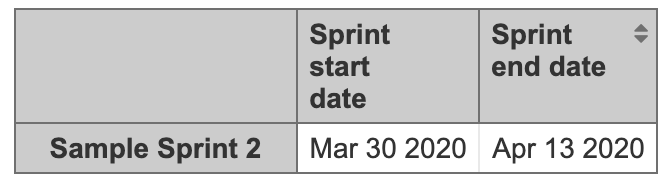# [RESOLVED] Problem in Filter Time Spent logged in current sprint

Good afternoon,

I have a problem, and I’ve looked for a lot of solutions and found nothing.

I need to list the total hours logged in the issues of a given Sprint, but I need to know only the hours that were logged between the day of the Start Sprint and the Closing day of the Sprint.

Sprint 09 - Start Date = 3/15/2020 | End Date = 03/30/2020
Sprint 10 - Start Date = 01/04/2020 | End Date = 4/14/2020

Example
Story A - 10 hours logged on 16/03/2020
Story A - 5 hours logged on 02/04/2020
Story B - 10 hours logged on 29/03/2020
Story B - 3 hours logged on 11/04/2020

On my dashboard, I need to list the sum of all hours logged in the sprint as follows:

Sprint 09 | 20 hours
Sprint 10 | 9 hours

Below is a real example.

I have 3 Issues in my “Sample Sprint 2” Sprint with a total of 22 logged hours

Bellow the Start and End date of the SprintBelow is the date of each time log of my Sprint Issues. Note that the Log highlighted in Red (10 hours), was made outside the period of my Sprint, so my measure should not return these 10 hours.

I found the following code:

``````Sum (Filter (
Descendants ([Time] .CurrentHierarchyMember, [Time]. [Day]),
[Measures]. [Time within Sprint]> 0
), [Measures]. [Hours spent with sub-tasks])
``````

However, not worked from me.

Can anyone help me with this problem?

Hi @lincoln19922,
I will start with your first example that I tried to reproduce but with the scenario you provided it looks that Hours spent together with Sprint works exactly as you would expect it.
Here is an example where I used precisely the date you mentioned and when the hours were logged.

In the second example, it looks that the issue was already added to the Sprint when hours were logged, and only after that, the Sprint was started. In this case, hours are counted into the Sprint and you need to create a calculated measure in Measure dimension that you already mentioned above. Tested with my example and it works like a charm where the measure Hours spent within the Sprint takes into account only hours logged after Sprint start date.